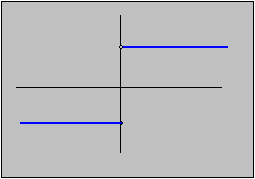#Interactive Real Analysis

Next | Previous | Glossary | Map

## 6.4. Topology and Continuity

### Examples 6.4.2(b):

Let f(x) = 1 if x > 0 and f(x) = -1 if x0. Show that f is not continuous by
1. finding an open set whose inverse image is not open.
2. finding a closed set whose inverse image is not closed.f(x) = 1 if x > 0 and f(x) = -1 if x0
The inverse image of the set (-2, 0) is the negative real axis, together with the origin. That set is closed. We have found an open set whose inverse image is not open; therefore the function is not continuous.

The inverse image of the set [0,2] is the positive real axis without the origin. That set is open. We have found a closed set whose inverse image is not closed; therefore, the function is not continuous.

Next | Previous | Glossary | Map